# HyperLogLog in Practice: Algorithmic Engineering of a State of the Art Cardinality Estimation Algorithm

Continuing on the theme of approximations from yesterday, today’s paper looks at what must be one of the best known approximate data structures after the Bloom Filter, HyperLogLog. It’s HyperLogLog with a twist though – a paper describing HyperLogLog in practice at Google and a number of enhancements they made to the base algorithm to make it work even better.

At Google, various data analysis systems such as Sawzall, Dremel and PowerDrill estimate the cardinality of very large data sets every day, for example to determine the number of distinct search queries on google.com over a time period. Such queries represent a hard challenge in terms of computational resources, and memory in particular: For the PowerDrill system, a non-negligible fraction of queries historically could not be computed because they exceeded theavailable memory. In this paper we present a series of improvements to the HyperLogLog algorithm by Flajolet et. al. that estimates cardinalities efficiently. Our improvements decrease memory usage as well as increase the accuracy of the estimate significantly for a range of important cardinalities.

In PowerDrill (and other database systems), a user can count the number of distinct elements in a data set by issuing a count distinct query. Such a query often contains a group-by clause meaning that a single query can lead to many count distinct computations being carried out in parallel. PowerDrill performs about 5 million such computations, 99% of these yielding a result of 100 or less. About 100 computations a day yield a result greater than 1 billion though. The key requirements at Google are therefore:

• As accurate an estimate as possible within a fixed memory budget, and for small cardinalities the result should be near exact.
• Efficient use of memory
• The ability to estimate large cardinalities (1 billion+) with reasonable accuracy
• The algorithm should be (easily) implementable and maintainable

As a quick refresher, the basis of HyperLogLog is hashing every item to be counted, and remembering the maximum number of leading zeros that occur for each hash value. If the bit pattern starts with d zeros, then a good estimate of the size of the multiset is 2d+1. Instead of relying on a single measurement, variability is reduced by splitting the input stream of data elements S into m substreams of roughly equal size by using the first p bits of the hash values (m=2p). Therefore we count the number of leading zeros after the first p bits. The final cardinality estimate is produced from the substream estimates as the normalised bias corrected harmonic mean: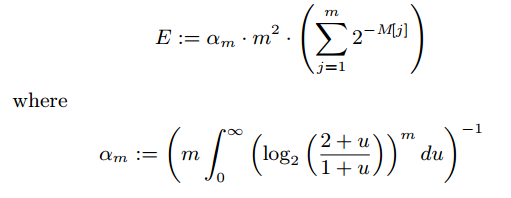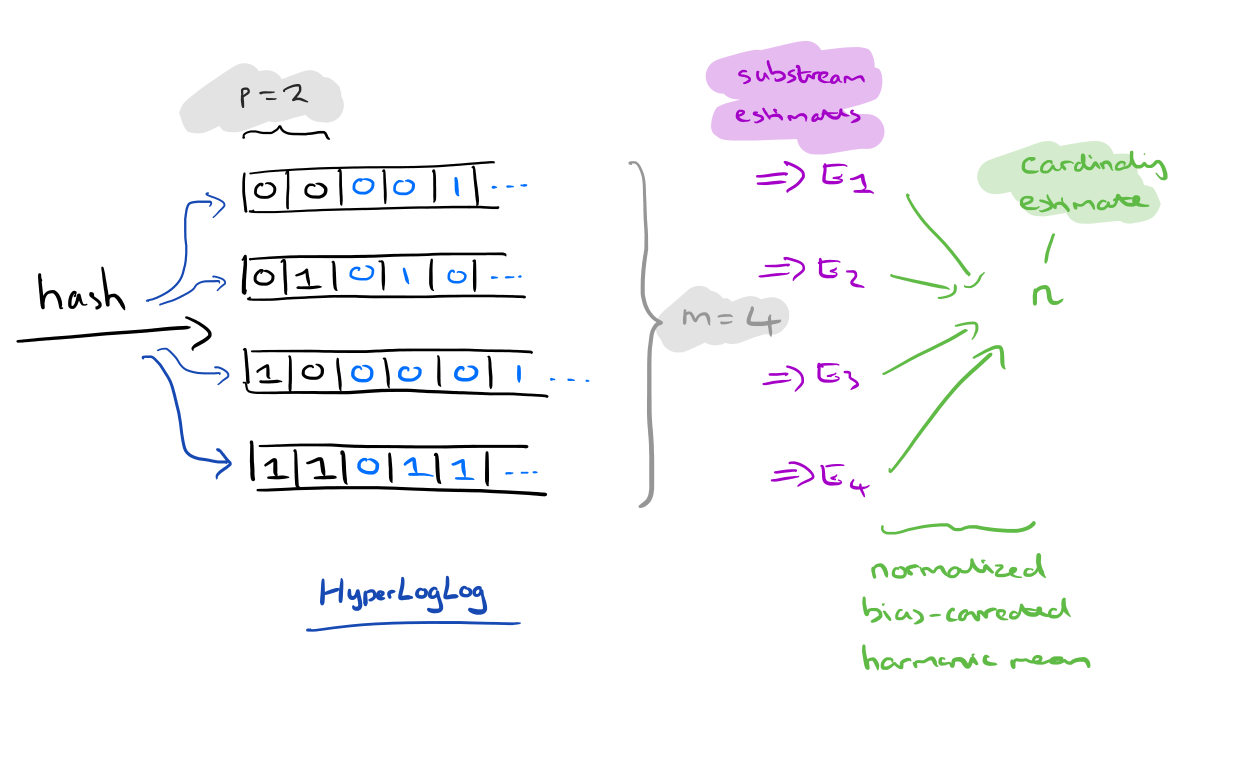For n < 5m/2 nonlinear distortions appear that need to be corrected, so in this range linear counting (m log(m/V), where V is the number of zeros) is used instead.

Google improve the base HyperLogLog algorithm in three areas: ability to estimate very large cardinalities; improved accuracy for small cardinalities; and reduction in the amount of space required.

### Estimating Large Cardinalities

A hash function of L bits can distinguish at most 2L values, and as the cardinality n approaches 2L hash collisions become more and more likely and accurate estimation becomes impossible. Google’s solution here is rather straightforward – use a 64-bit hash function in place of a 32-bit one. We don’t actually need to store the hashes themselves, just the maximum size of the number of leading zeros + 1, so 64-bit hashes only increases the memory requirement from 52p bits to 62p bits.

### Improving Accuracy for Small Cardinalities

Recall that for n < 5m/2 the raw estimate of HyperLogLog is inaccurate, and linear counting is used instead. In the chart below, the red line shows the raw HLL estimate, and the green line shows the more accurate estimate produce by linear counting for small n.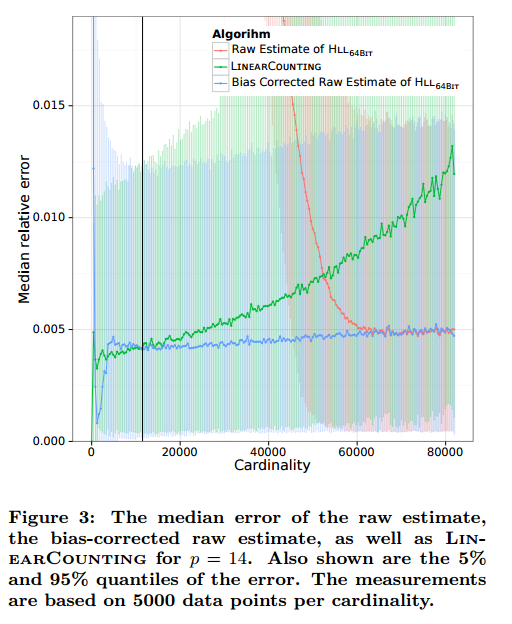The blue line on the above chart shows an improved estimate for small n that gives even better accuracy. This estimate is produced by directly correcting for bias in the original raw estimate.

In simulations, we noticed that most of the error of the raw estimate is due to bias; the algorithm overestimates the real cardinality for small sets. The bias is larger for smaller n, e.g., for n = 0 we already mentioned that the bias is about 0.7m. The statistical variability of the estimate, however, is small compared to the bias. Therefore, if we can correct for the bias, we can hope to get a better estimate, in particular for small cardinalities.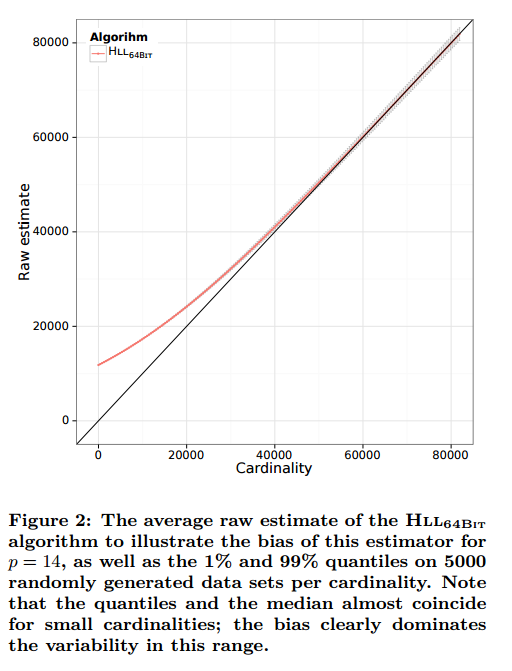To determine the bias, the authors calculate the mean of all raw estimates for a given cardinality, minus that cardinality.

With this data, for any given cardinality we can compute the observed bias and use it to correct the raw estimate. As the algorithm does not know the cardinality, we record for every cardinality the raw estimate as well as the bias so that the algorithm can use the raw estimate to look up the corresponding bias correction. To make this practical, we choose 200 cardinalities as interpolation points, for which we record the average raw estimate and bias. We use nearest neighbor interpolation to get the bias for a given raw estimate (for k = 6).

Notice in the first figure of this section, that linear counting still beats the bias-corrected estimate for small n. For precision 14 (p=14) the error curves intersect at around n = 11,500. Therefore linear counting is used below 11,500, and bias corrected raw estimates above.

The end result is that for an important range of cardinalities – roughly between 18,000 and 61,000 when p=14 , the error is much less than that of the original HyperLogLog.

### Saving Space

In the original algorithm we maintain an array M of registers of size m = 2p. Each registers holds 1 + the maximum number of leading zeros seen so far for the corresponding hash prefix. If n << m then most of the registers are never used. In this case we can use a sparse representation that stores (index,register-value) pairs. If the list of pairs requires more memory than the dense representation of the registers (the array M), then the list can be converted to the dense representation.

In our implementation, we represent (index, register-value) pairs as a single integer by concatenating the bit patterns… Our implementation then maintains a sorted list of such integers. Furthermore, to enable quick insertion, a separate set is kept where new elements can be added quickly without keeping them sorted. Periodically, this temporary set is sorted and merged with the list.

When only the sparse representation is used, accuracy can be increased by choosing a different precision argument p’ > p. If the sparse representation gets too large and needs to be converted to a dense representation, then it is possible to fall back to the lower precision p.

In the sparse implementation, we can use a more efficient representation for the list itself too…

We use a variable length encoding for integers that uses a variable number of bytes to represent integers, depending on their absolute value. Furthermore, we use a difference encoding, where we store the difference between successive elements in the list. That is, for a sorted list a1,a2, a3, … we would store a1, a2-a1, a3-a2, … The values in such a list of differences have smaller absolute values, which makes the variable length encoding even more efficient.

The cutoff point between the sparse and dense representations is such that we know that the linear counting method will always be used to produce the cardinality estimation. This fact can be taken advantage of to yield a further space saving – see section 5.3.3 in the paper for details.

### Comparison

The final Google algorithm with all the improvements is denoted HLL++. The following chart compares the accuracy of the original HLL algorithm with HLL++.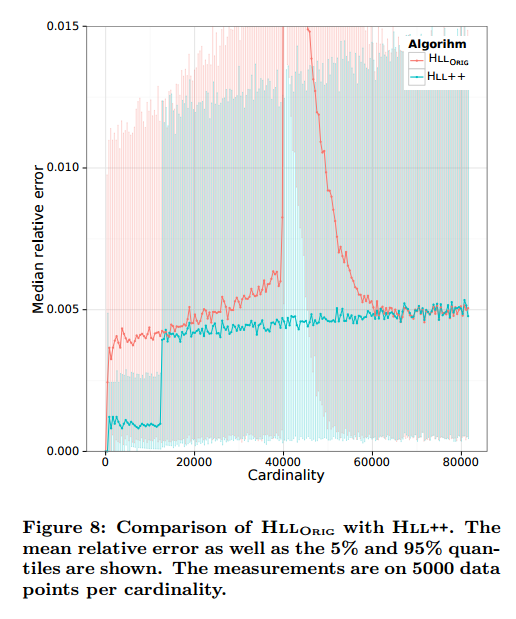All of these changes can be implemented in a straight-forward way and we have done so for the PowerDrill system. We provide a complete list of our empirically determined parameters at http://goo.gl/iU8Ig to allow easier reproduction of our results.